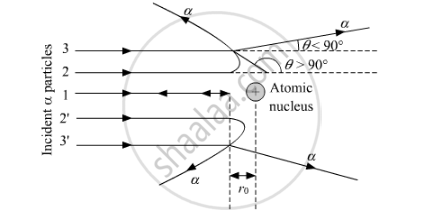# In the Study of Geiger-marsdon Experiment on Scattering of α Particles by a Thin Foil of Gold, Draw the Trajectory of α-particles in the Coulomb Field of Target Nucleus. Explain Briefly How One Gets the Information on the Size of the Nucleus from this Study. - Physics

In the study of Geiger-Marsdon experiment on scattering of α particles by a thin foil of gold, draw the trajectory of α-particles in the coulomb field of target nucleus. Explain briefly how one gets the information on the size of the nucleus from this study.

From the relation R = R0 A1/3, where R0 is constant and A is the mass number of the nucleus, show that nuclear matter density is independent of A

#### Solution

Trajectory of α Particles in Coulomb Field of Target NucleusFrom this experiment, the following is observed:
1. Most of the alpha particles pass straight through the gold foil. It means that they do not suffer any collision with gold atoms.

2. About one alpha particle in every 8000 alpha particles deflects by more than 90°

As most of the alpha particles go undeflected and only a few get deflected, this shows that most of the space in an atom is empty and at the centre of the atom, there exists a nucleus. By the number of the alpha particles deflected, the information regarding size of the nucleus can be known.

If m is the average mass of a nucleon and R is the nuclear radius, then mass of nucleus = mA, where A is the mass number of the element.

Volume of the nucleusV=4/3piR^3

=>V=4/3pi(R_0A^"1/3")^3

=>V=4/3piR_0^3A

Density of nuclear matterrho=(mA)/V

=>rho=(mA)/(4/3R_0^3A)

=>rho=(3m)/(4piR_0^3

This shows that the nuclear density is independent of A

Concept: Atomic Masses and Composition of Nucleus
Is there an error in this question or solution?
2014-2015 (March) Delhi Set 1

Share